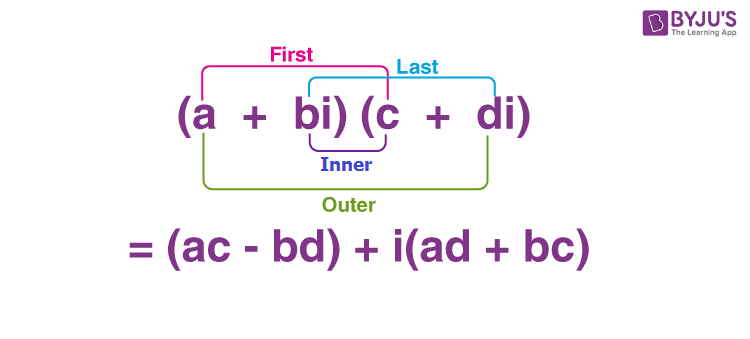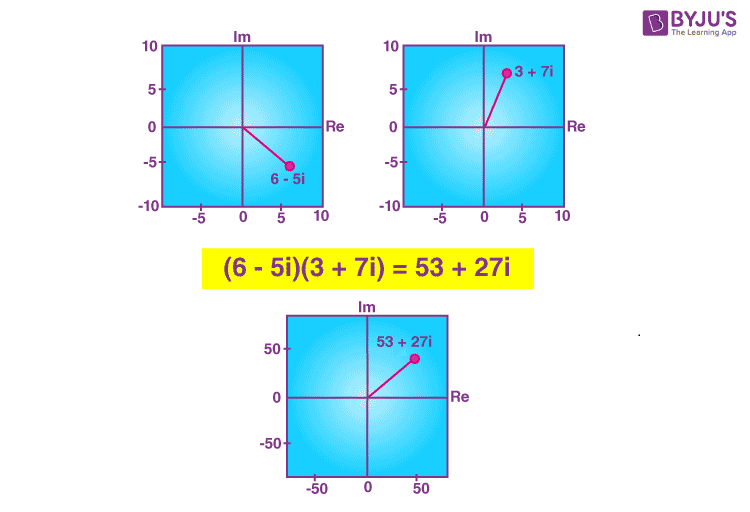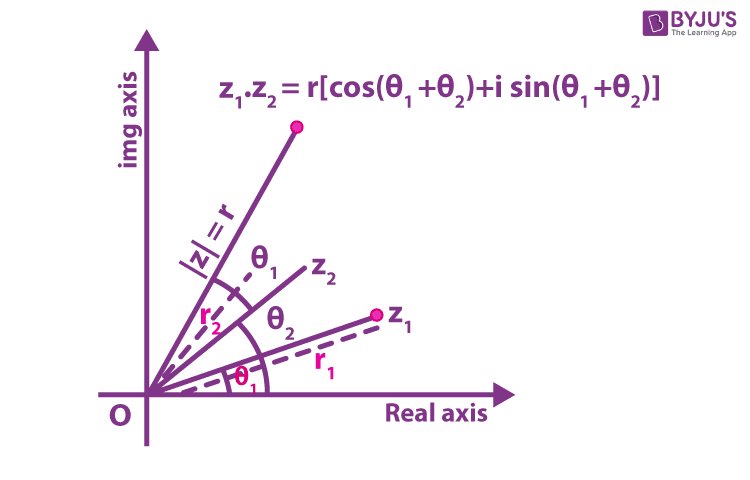# Multiplication of Complex Numbers

Before getting into the multiplication of complex numbers, let’s have a recall on what is a complex number and how to represent it. In mathematics, we can write the complex numbers in the form z = x + iy, where x and y are real numbers, and i is a symbol called the imaginary unit, and satisfying the equation i2 = −1. No “real” number satisfies this equation and i was called an imaginary number by René Descartes. As we know, x is the real part and y is called the imaginary part for the complex number x + iy. Either of the symbols $$\mathbb{C}$$ or C denotes the set of complex numbers.

Unlike numbers, it is also possible to perform algebraic operations on complex numbers. In this article, you will learn how to perform multiplication on complex numbers along with geometrical representations.

## Multiplication of Complex Numbers Formula

Suppose z1 = a + ib and z2 = c + id are two complex numbers such that a, b, c, and d are real, then the formula for the product of two complex numbers z1 and z2 is derived as given below:

Go through the steps given below to perform the multiplication of two complex numbers.

Step 1: Write the given complex numbers to be multiplied.

z1z2 = (a + ib)(c + id)

Step 2: Distribute the terms using FOIL technique to remove the parentheses.

z1z2 = ac + i(ad) + i(bc) + i2(bd)

Step 2: Simplify the powers of i and apply the formula i2 = –1.

z1z2 = ac + i(ad) + i(bc) + (-1)(bd)

Step 3: Combine like terms that means combine real numbers with real numbers and imaginary numbers with imaginary numbers.

z1z2 = (ac – bd) + i(ad + bc)

### Multiplication of Complex Numbers by FOIL Method## Multiplication of Two Complex Numbers

Algebraically, the multiplication of two complex numbers can be found using the formula derived above. Let’s understand this with the help of an example given below:

Example:

Find the product of complex numbers (6 – 5i) and(3 + 7i).

Solution:

(6 – 5i)(3 + 7i) = (6) × (3) + (6) × (7i) – (5i) × (3) – (5i) × (7i)

= 18 + 42i – 15i – 35i2

= 18 + 27i – 35(-1)

= 53 + 27i

### Geometrical Representation of Multiplication of Complex Numbers

We can represent the multiplication or product of two complex numbers geometrically in the argand plane. For the above example, the geometrical representation is given in the below figure:### Multiplication of Complex Numbers in Polar Form

Suppose z1 = r1(cos θ1 + i sin θ1) and z2 = r2(cos θ2 + i sin θ2) are two complex numbers in polar form, then the product, i.e. multiplication of these two complex numbers can be found using the formula given below:

z1z2 = r1 r2 [cos (θ1 + θ2) + i sin (θ1 + θ2)]

Geometrically we can show the multiplication of polar form of complex numbers as:### Properties of Multiplication of Complex Numbers

If z1, z2, and z3 are any three complex numbers, then the following properties can be defined.

• The closure law The product of two complex numbers is a complex number, the product z1z2 is a complex number for all complex numbers z1 and z2.
• Commutative law on multiplication of two complex numbers: z1z2 = z2z1
• Associative law of multiplication of complex numbers: (z1z2)z3 = z1(z2z3)
• The existence of multiplicative identity: There exists the complex number 1 + i 0 (denoted as 1), called the multiplicative identity such that z.1 = z, for every complex number z.
• The existence of multiplicative inverse: For every non-zero complex number z = x + iy, we have the complex number [x/(x2 + y2)] – i[y/(x2 + y2)] (denoted by z−1 or 1/z) such that

z.(1/z) = (1/z).z = 1

Here, 1/z is called the multiplicative inverse of z.

• Multiplication of complex numbers is distributive over the addition of complex numbers.
• The distributive law: For any three complex numbers z1, z2 and z3

z1(z2 + z3) = z1z2 + z1z3

(z1 + z2)z3 = z1z3 + z2z3

## Multiplication of Complex Numbers Examples

Go through the solved examples given below for better understanding of the concept.

Example 1: Compute (7 + 2i)(4 – 6i)

Solution:

(7 + 2i)(4 – 6i)

Using the formula (a + ib)(c + id) = (ac – bd) + i(ad + bc),

a = 7, b = 2, c = 4, d = -6

(7 + 2i)(4 – 6i) = (7)(4) – (2)(-6) + i[(7)(-6) + (2)(4)]

= 28 + 12 + i(-42 + 8)

= 40 – 34i

Example 2: Find the product of three complex numbers (3 + 4i), (2 – 3i) and (5 + 4i).

Solution:

(3 + 4i)(2 – 3i)(5 + 4i)

= [(3)(2) – 3(3i) + (4i)(2) – (4i)(3i)] (5 + 4i)

= [6 – 9i + 8i – 12i2] (5 + 4i)

= [6 – i – 12(-1)](5 + 4i)

= (18 – i)(5 + 4i)

= (18)(5) + 18(4i) – 5i – (i)(4i)

= 90 + 72i – 5i – 4i2

= 90 + 67i – 4(-1)

= 90 + 67i + 4

= 94 + 67i

To get more articles related to complex numbers and arithmetic operations on complex numbers, visit www.byjus.com today!

## Frequently Asked Questions on Multiplication of Complex Numbers

### What is the product of two complex numbers?

The product or multiplication of two complex numbers is also a complex number. That means, the product of two complex numbers can be expressed in the standard form A + iB where A and B are real.

### How do you multiply complex numbers?

Suppose z1 = a + ib and z2 = c + id are two complex numbers, then the multiplication or product of these two complex numbers can be calculated using the formula z1z2 = (ac – db) + i(ad + bc).

### What is the multiplicative identity of complex numbers?

There exists the complex number 1 + i 0 (denoted as 1), called the multiplicative identity such that z.1 = z, for every complex number z.

### What properties apply to multiplication with complex numbers?

The following properties can be defined for the multiplication of complex numbers:
Closure law
Commutative law
Associative law
Distributive law
The existence of multiplicative identity
The existence of multiplicative inverse

### How do you simplify the product of complex numbers?

We can simplify the product of complex numbers using FOIL technique.

### Is the complex number closed under multiplication?

Yes, complex numbers are closed under multiplication.